# RSI Period Length Effect

## RSI Period Settings

The period length parameter decides how stable or volatile RSI will be and how fast it will react to changing market conditions (up and down moves of new bars).

Below you can find a few RSI charts (source: RSI Calculator). All charts are for the same market (daily data of S&P500 index). The only difference is the RSI period length. You can see how the RSI behaves differently based on the period length setting.

The most popular RSI period setting is 14: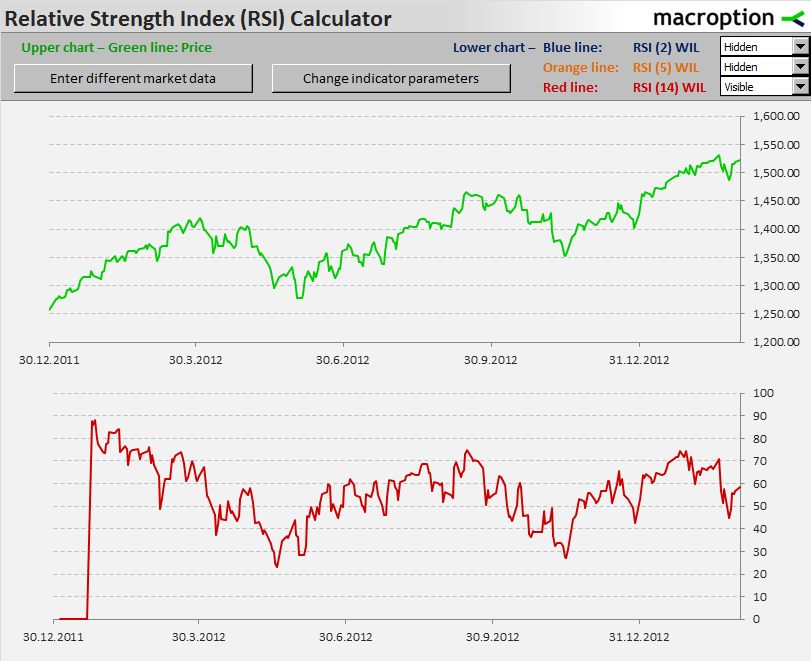When you set a shorter period, the RSI will reach extreme values (above 80 or below 20) more often. Overall it will appear more volatile or unstable. A chart for the same data with RSI 5 is below: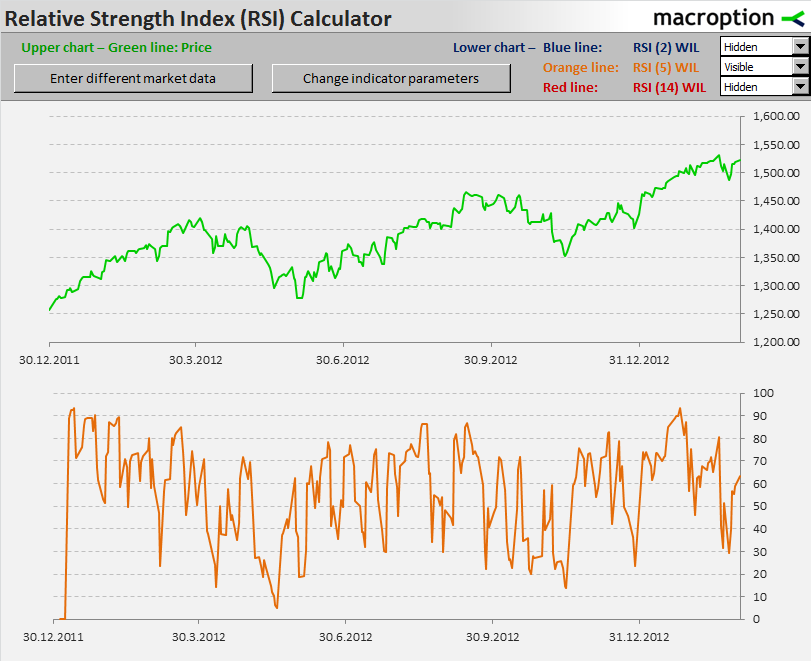RSI with period of 2. You can see that the RSI goes almost to 0 or 100 quite often: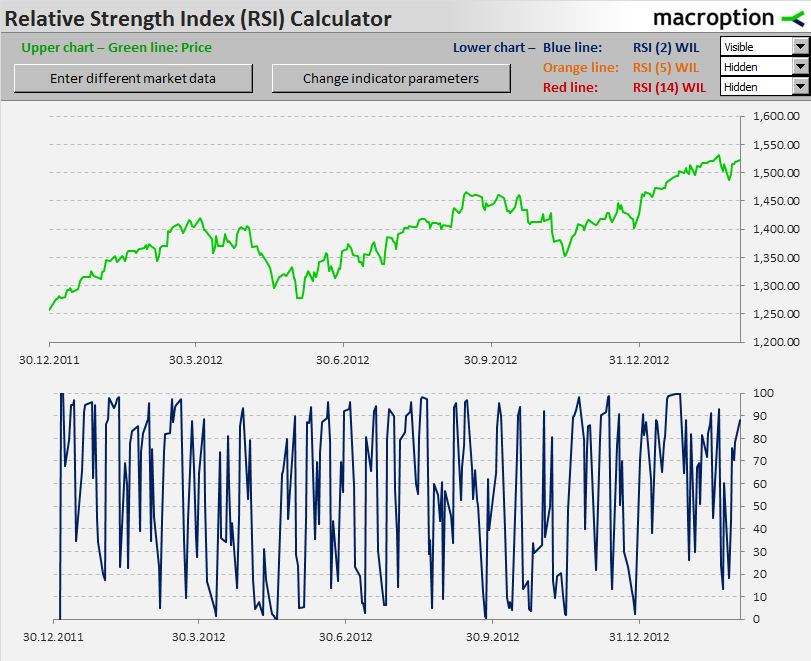Here you can see all three settings in one chart: RSI with period equal to 2 (blue), 5 (orange), and 14 (red):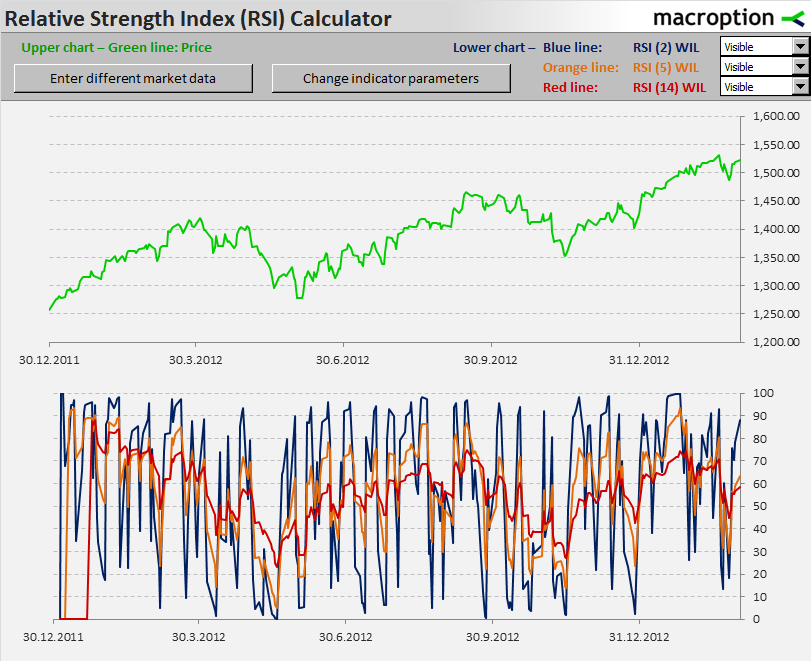The longer the RSI period, the smoother or more stable the RSI chart. Below you can see one more chart with RSI 14 (red) and RSI 30 (blue):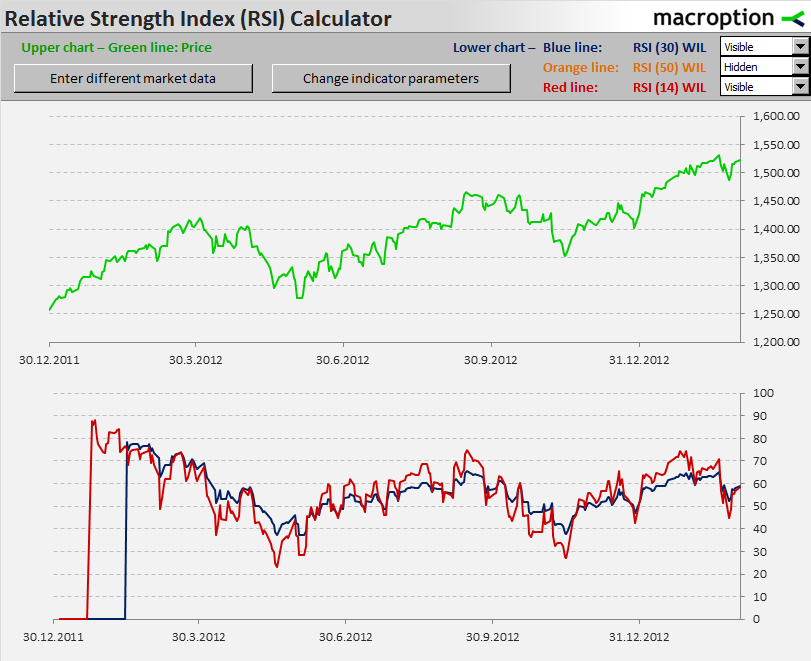And one more with RSI 50. You can see that now the RSI rarely leaves the centre of the value range around 50: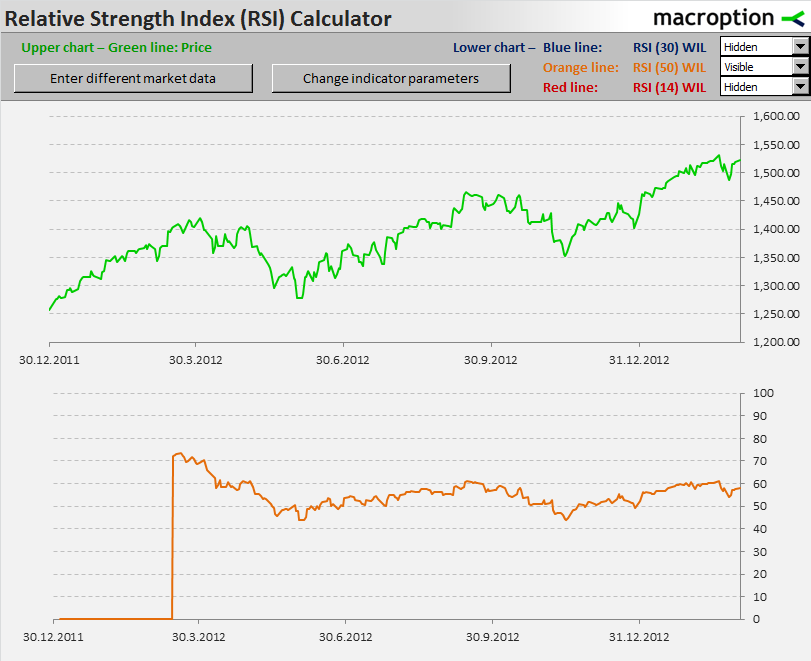## Effect of Period Length Based on Average Method

The effect of period length is different under different RSI calculation methods. The general rule is always the same (shorter period means more volatile RSI), but there are little details in the effects of particular period values on RSI values. This is also quite obvious from the formulas.

In fact, the parameter exactly represents the number of bars used in the calculation under the Simple MA method only.

Under the other two methods the period length parameter decides how fast the importance of individual bars diminishes going from the current bar to older bars, but the current RSI is affected by all previous bars since the very beginning of the data. Although the weight of the bars long time ago is negligible, it is not zero. This also means that Exponential MA and Wilder smoothing can result in slightly different RSI values with the same settings but different start of data.

## How to Choose RSI Period

Like with the averaging methods, there is no “right” or “best” period and being consistent is more important than the exact number itself. The selection should be based on your data, time horizon, trading style, and purpose of using RSI in general.

The most popular RSI period is 14, which is also what J. Welles Wilder Jr. suggests in his book (pages 63-70).

The RSI Calculator allows you to put up to 3 different RSI indicators on the chart at the same time, so you can compare how different settings looks in the same situation (for actual trading it is better to use just one, maybe two indicators simultaneously). Further explanation of RSI calculation and practical use is available in the calculator’s PDF guide.

Have a question or feedback? It takes less than a minute.